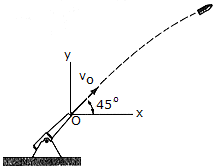# Engineering Mechanics - KOP: Impulse and Momentum - Discussion

### Discussion :: KOP: Impulse and Momentum - General Questions (Q.No.9)

9.The projectile having a mass of m = 3 kg is fired from a cannon with a muzzle velocity of vO = 500 m/s. Determine the projectile's angular momentum about point O at the instant it is at the maximum height of its trajectory.

 [A]. H0 = 15.11(106) kg- m2/s CCW [B]. H0 = 6.76(106) kg- m2/s CCW [C]. H0 = 15.11(106) kg- m2/s CW [D]. H0 = 6.76(106) kg- m2/s CW

Explanation:

No answer description available for this question.